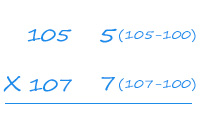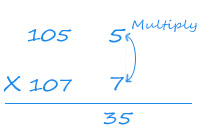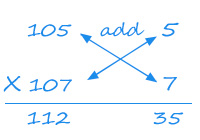More
BlogMath3 Ways to Multiply Numbers Just Over 100 in 3 Seconds

# 3 Ways to Multiply Numbers Just Over 100 in 3 SecondsHow fast can you calculate 105 X 107?

Ah! searching for your calculator?

In part 1 of this series of posts, SplashLearn Wizard will let you in on a cool trick to perform such multiplications mentally! You would not need a calculator at all!

The answer will unfold in 3 simple steps:

Step 1:  Subtract 100 from the numbers and write the differences besides the numbers. (Look at the following illustration)Step 2: Now multiply the differences i.e. 5 x 7 = 35, and write the product down.Step 3: Add numbers diagonally, i.e. 105 + 7 or 107 + 5 ( sum will be same ). Write the result of addition on the left hand side.And Voila! we have multiplied two three digit number in a matter of seconds.

We have explained the procedure in 3 steps, but if you think this can be done mentally.

Try multiplying :

a. 102 x 103

b. 109 x 108

However, there is a catch. This trick works for numbers just over 100 and less than 110.

In our future posts we will teach you to take it to the next level. Keep coming back to learn more tricks.

Now go and impress your friends 🙂

AUTHOR